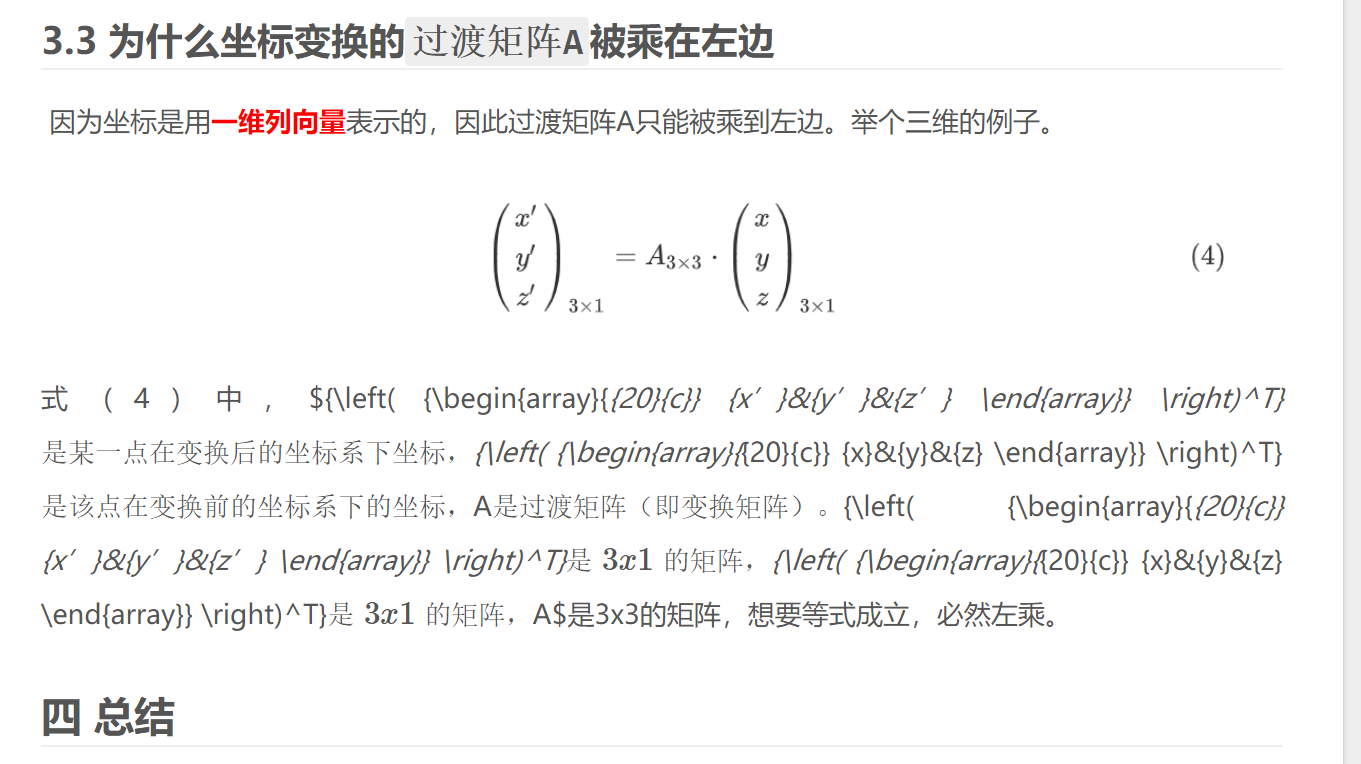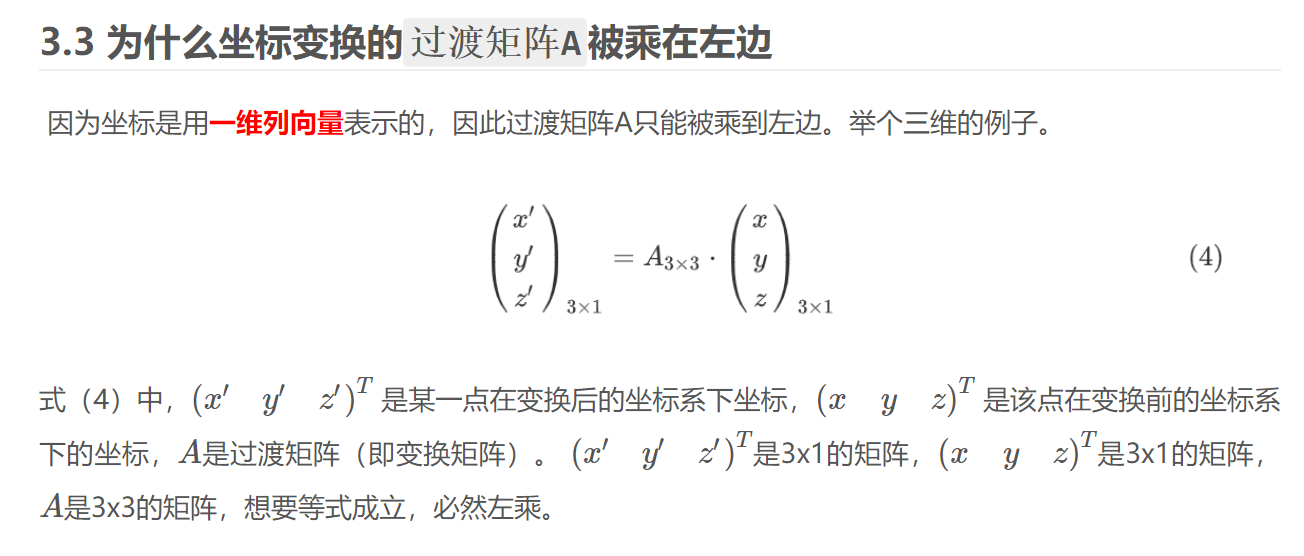• 长公式换行用\，对齐用&，宏包\usepackage{amsmath} 代码示例 \begin{equation*}%加*表示不对公式编号 \begin{split} PMV =&[0.303*exp(-0.036M)+0.0275]*\{M-W-3.05*[5.733-\\ &0.007(M-W)-P_a]-0.42*...
长公式换行用\，对齐用&，宏包\usepackage{amsmath}
代码示例
\begin{equation*}%加*表示不对公式编号
\begin{split}
PMV =&[0.303*exp(-0.036M)+0.0275]*\{M-W-3.05*[5.733-\\
&0.007(M-W)-P_a]-0.42*(M-W-58.2)-0.0173M*\\
&(5.867-P_a)-0.0014M*(34-t_a)-3.96*10^-8*f_cl*[(t_cl+\\
&273)^4-(t_r+273)^4]-f_cl*h_c*(t_cl-t_a)\}
\end{split}
\end{equation*}



展开全文• 1.导入宏包\usepackage{stfloats}。 2. \newcounter{TempEqCnt} % 创建临时变量TempEqCnt \setcounter{TempEqCnt}{\value{...\setcounter{equation}{15} % 当前公式序号变为x，x等于长公式应有的序号减1. \begin{fi
转自https://blog.csdn.net/lma_hzq/article/details/104438668
1.导入宏包\usepackage{stfloats}。
2.
\newcounter{TempEqCnt} % 创建临时变量TempEqCnt
\setcounter{TempEqCnt}{\value{equation}} % 将当前公式序号 赋给TempEqCnt
\setcounter{equation}{15} % 当前公式序号变为x，x等于长公式应有的序号减1.
\begin{figure*}[ht] %hb代表放在文章底部，%ht为放在文章顶部
\begin{equation}
公式（16）
\end{equation}
\begin{equation}
公式（17）
\end{equation}
\begin{equation}
公式（18）
\end{equation}
\end{figure*}

3.注意事项：公式会跑到文章的后一页，要想放在当前页需要在上一页代码处进行插入，但是这么操作后面的公式序号会乱，需要自定义后面公式的序号。


展开全文• 在使用hexo的next主题的latex公式时，行间如果公式太，则容易出现个人博客显示不全公式的问题。 1. 笔记markdown编辑页面 2. 个人博客中表现 解决 行间公式用$$公式$$，不要用$公式$ ...
问题描述
在使用hexo的next主题的latex公式时，行间如果公式太长，则容易出现个人博客显示不全公式的问题。
1. 笔记markdown编辑页面2. 个人博客中表现解决
行间公式用$$公式$$，不要用$公式$

2020-5-27表明：除了上面的问题，latex公式中多个左括号黏在一起会报错，应该用空格把他们分开。
{{x+y}}   这是错误的
{ {x+y}}  这是正确的展开全文markdown
• ## LaTex公式长度过长

千次阅读 2019-12-18 14:31:44
以下几种方法都有自己的适用...原来的公式： \begin{equation} this is a very long equation. \end{equation} 修改后的公式： \begin{equation} \begin{aligned} this is & a very \\ long & equation....
以下几种方法都有自己的适用场景，我们可以根据自己的实际情况酌情选择：
方法一：断行
原来的公式：
\begin{equation}
this is a very long equation.
\end{equation}

修改后的公式：
\begin{equation}
\begin{aligned}
this is & a very \\
long & equation.\\
\end{aligned}
\end{equation}

方法二：适量缩小公式间距  微调公式长度
原来的公式：
\begin{equation}
this is a very long equation.
\end{equation}

修改后的公式：
\begin{equation}
this \! \! is \!\! a  \! very  \! long  \!  equation.
\end{equation}

方法三：变小字体
原来的公式：
\begin{equation}
this is a very long equation.
\end{equation}

修改后的公式：
{\small
\begin{equation}
this is a very long equation.
\end{equation}
}

除了\small 之外，大小依次递减还可以选择\footnotesize，\scriptsize。


展开全文• LATEX公式下标短横线过长 我其实也不太知道为什么，但是我想应该可以定义破折号的长度，所以我百度了定义破折号长度的方法。然后将raisebox改为零，得到： $G_{s\raisebox{0mm}{-}t}$ 就好了。
• LaTeX公式过长影响显示的解决办法 在写论文的时候，发现如果写双栏论文，公式过长的时候，会影响到另一栏的显示 ，即会出现以下尴尬情况： 为了解决这个问题，尝试了两种方法，记录如下： 1.对单个公式进行...缩放
• 当你的Latex公式刚好那么一点点 今天在写论文的时候遇见了一个让我非常抓狂的情形：双栏排列的情况下，有一个式子刚好比半页幅面了那么一点点，如下： ar(k)=[1⋯ej(m1Ψkr1+m2Ψkr2)⋯ej[(M1−1)Ψkr1+(M2−1...长度
• latex公式过长通常有以下几个解决方案： （1）使用amsmath package的split环境 \begin{equation} \begin{split} F = \{F_{x} \in F_{c} &: (|S| > |C|) \\ &\quad \cap (\text{minPixels} &\quad \cap (|S_{\text...
• The gutter between columns is 0.155 inches wide (on page 4), but should be at least 0.2 inches 如果是由于公式长度超过文本长度，且公式不适宜换行，那么可以在等号左右加上\!，缩小等号左右的距离 插入前： ...
• ## LaTeX公式

万次阅读 2018-08-31 22:04:51
LaTeX公式 行内公式 -$公式$ 行间公式 -$$公式$$ 使用需要用到的包 \usepackage{mathrsfs} \usepackage{amsmath} \newcommand \newcommand{\fr}{\frac} % 类似于宏定义，将\frac 用\fr 表示 \...
• latex公式进行换行 \begin{equation} \begin{aligned} &Ttran_{i}^{j,j+1}=\frac{R_{bd_{j,j+1}}^{ij}}{AR_{bd_{j,j+1}}^{ij}}\times T_{ideal\_tran}^{ij},\\ &v,u \in \left [ 1,|E_{S}| \right ],j,j+1...
• LaTeX公式与MathType公式的快速转换abstract1. LaTeX在线公式编辑器：2. MathType公式编辑器：3. MathType公式转LaTex公式：3.1 例：MathType公式转LaTex公式4. LaTex公式转MathType公式：4.1 例LaTex公式转MathType...MathType math 数学
• ## LaTeX公式编辑

千次阅读 2018-04-12 22:01:11
LaTeX多行公式显示一个编号LaTeX公式中有大括号
• 首先一定要插入两个包： \usepackage{amsmath} \usepackage{amssymb} ...然后文章中可如下编译公式： \begin{equation}\label{1} \begin{aligned} a & = b + c \\ & = d + e \end{aligned} \end{equa...
• ## latex公式标号测试

千次阅读 2019-01-07 14:14:52
• LaTeX 公式过长怎么办： 方法一，缩小公式，前后加\small； $xyz$ xyzxyzxyz $\small xyz \small$ xyz\small xyz \smallxyz 方法二，分行 D&=D_1+D_2+D_3+D_4+D_5\\ & +D_6+D_7+D_8 第一行等号前加&，行尾加\，第二...
• LaTeX公式转MathType公式步骤： 方式一： 打开链接，复制编写的LaTex公式，加入中，右键选择如图，复制代码到word的MathType公式编辑器里面即可。 https://www.zybuluo.com/mdeditor 方式二： 类似地，打开链接...
• 常用数学符号的 LaTeX 表示方法１、指数和下标可以用^和_后加相应字符来实现。比如：2、平方根（square root）的输入命令为：\sqrt，n 次方根相应地为: \sqrt[n]。方根符号的大小由LATEX自动加以调整。也可用\surd ...
• 需要对齐的长公式可以用split 环境，它本身不能单独使用，因此也称作次环境，必须包含在equation 或其它数学环境内。split 环境用 \ 和& 来分行和设置对齐位置。 \begin{equation} \begin{split} x=&a+b+c...
• ## LaTeX公式自动换行

千次阅读 2021-01-12 14:13:38
LaTeX公式自动换行 文章目录LaTeX公式自动换行前言一、autobreak宏包二、breqn宏包总结 前言 在使用amsmath等宏包输入公式的时候，最折腾的就是比较特殊样式的公式和公式，尤其是公式在投稿期刊排版的时候经常...
• 如果为了编辑这个公式而去学习LaTeX公式语法，那可就太蛋疼了！！！ 下面推荐一个在线可视化LaTeX公式编辑器，你可以利用里面的可视化工具编辑好公式，然后它会直接给你生成该公式的代码，到时候直接复制到Markdown...
• 曾经使用一个工具，可以将LaTeX数学公式直接转换成可以粘贴成word的公式，那个工具还有很多功能，但了几年忘了叫什么了，找到了一个在线转换的网站，可以供需要将LaTeX打好的数学公式放进word里的人参考。......